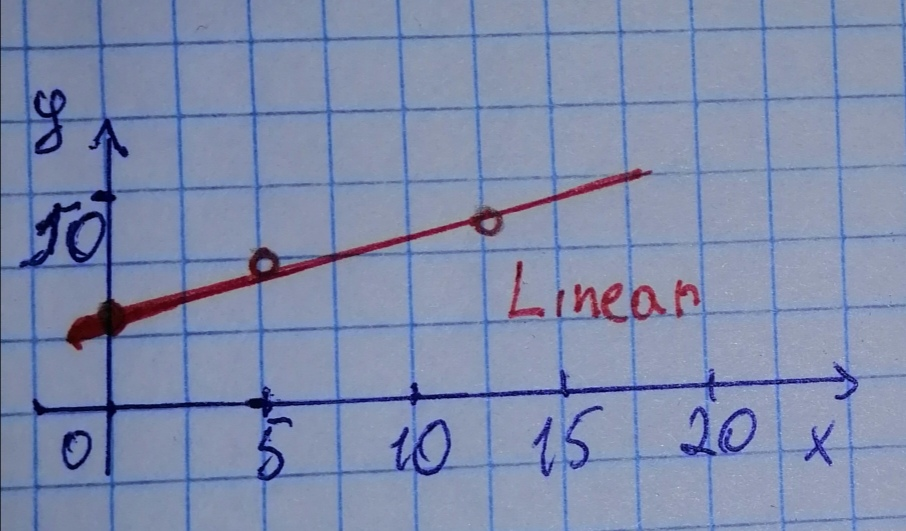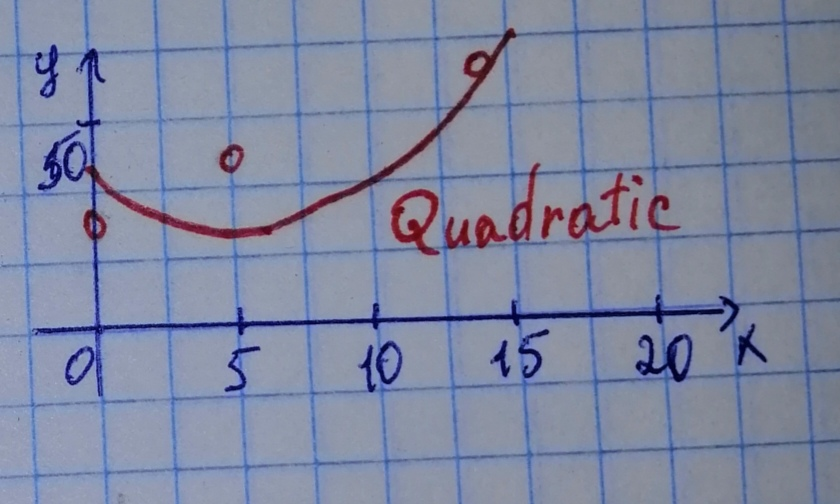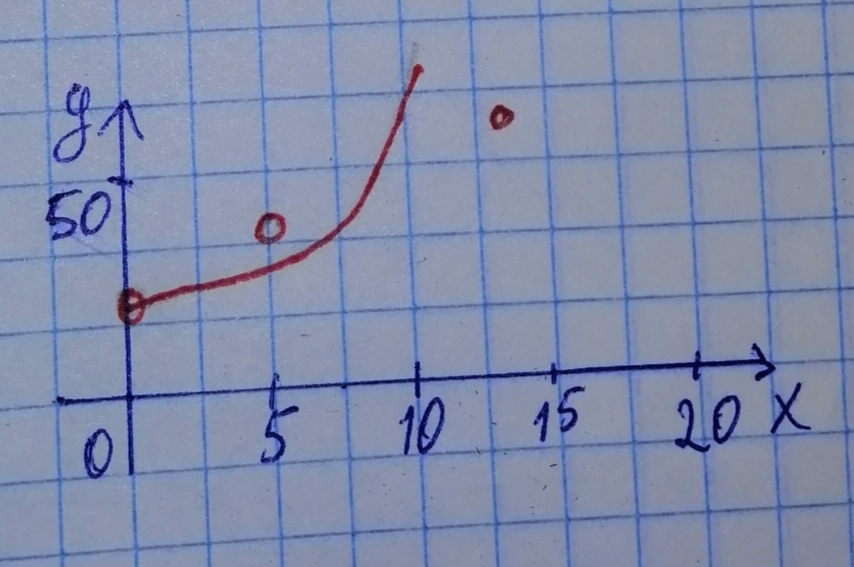# The following table shows the approximate average household income in the United States in 1990, 1995, and 2003.mattgondek4 2020-12-24 Answered

The following table shows the approximate average household income in the United States in 1990, 1995, and 2003. ($t=0$ represents 1990.)

Which of the following kinds of models would best fit the given data?
Explain your choice of model. ( a, b, c, and m are constants.)
a) Linear:
c) Exponential: $H\left(t\right)=A{b}^{t}$

You can still ask an expert for help

• Questions are typically answered in as fast as 30 minutes

Solve your problem for the price of one coffee

• Math expert for every subject
• Pay only if we can solve itTalisha

Step 1
Plot the points on acoordinate system.
Scetching the various models and their characteristics (see below), we find that the linear model would be best for this data set.Step 2
not exponential, because the rate of rising does not seem to change.
Not quadratic, because there are no "dips" or "bulges" to account for minimum/maximum values.